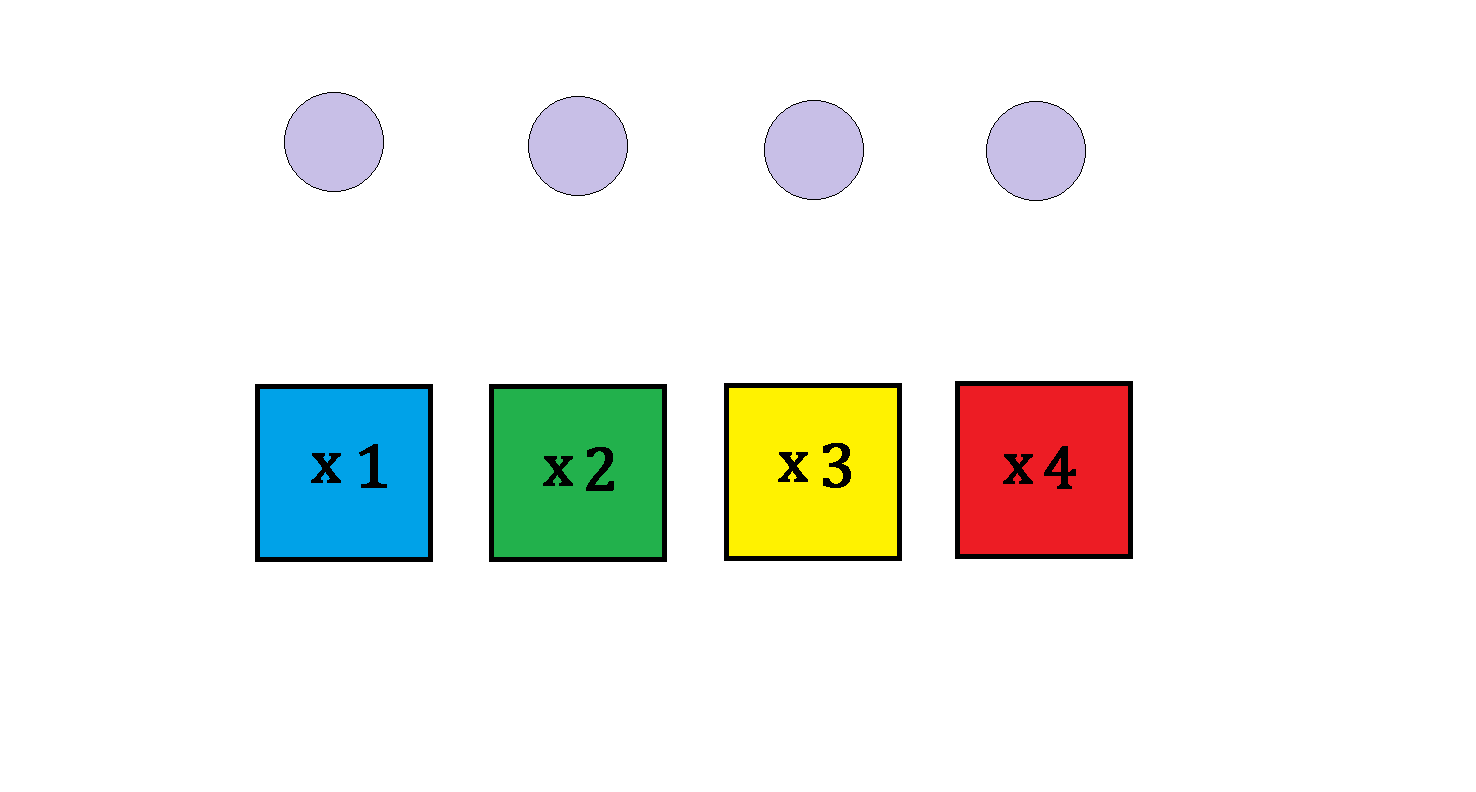# Magic Box Game

You're in a game show called "Magic Box," where you're given 4 identical balls to throw into $4$ boxes randomly. Each box will earn you the point(s) written on it multiplied by the number of balls thrown into it. For example, if you put one ball into each box, you'll get $1+2+3+4 =10$ points.

What is the expected value of points earned from this game?×